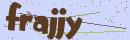# PHP开发薅羊毛信息提醒

2020-06-22 19:41:10

jike.info

yangmaoribao.com```<?php

\$url = 'https://jike.info/api/recent?_=';

\$lst_data = json_decode(file_get_contents(\$url), true)['topics'];   // jike.info

\$url = 'https://yangmaoribao.com/api/recent?_=';

\$lst_datas = json_decode(file_get_contents(\$url), true)['topics'];  // 羊毛日报

\$lst_data = array_merge(\$lst_data, \$lst_datas);

if (!\$lst_data) exit('error');

\$html = '';

\$one_hour_ago = (time() - 3600) * 1000;

foreach (\$lst_data as \$row_data) {
if (\$row_data['timestamp'] > \$one_hour_ago) {

\$post_time = date('Y-m-d H:i:s', \$row_data['timestamp'] / 1000);

\$url = "https://jike.info/topic/{\$row_data['slug']}";

if (mb_strpos(\$row_data['user']['picture'], 'yangmaoribao') !== false) {
\$url = "https://yangmaoribao.com/topic/{\$row_data['slug']}";
}

\$html .= "{\$row_data['title']} - {\$post_time} <br> <a href='{\$url}' target='_blank'>直达链接</a> <br><br>";
}
}

if (empty(\$html)) exit('error');

echo \$html;```

wxpusher.zjiecode.com/docs

http://wxpusher.zjiecode.com/docs/#/?id=%e6%b3%a8%e5%86%8c%e5%b9%b6%e4%b8%94%e5%88%9b%e5%bb%ba%e5%ba%94%e7%94%a8http://wxpusher.zjiecode.com/docs/#/?id=%e5%8f%91%e9%80%81%e6%b6%88%e6%81%af-1

```<?php

\$url = 'https://jike.info/api/recent?_=';

\$lst_data = json_decode(file_get_contents(\$url), true)['topics'];   // jike.info

\$url = 'https://yangmaoribao.com/api/recent?_=';

\$lst_datas = json_decode(file_get_contents(\$url), true)['topics'];  // 羊毛日报

\$lst_data = array_merge(\$lst_data, \$lst_datas);

if (!\$lst_data) exit('接口错误');

\$html = '';

\$one_hour_ago = (time() - 3600) * 1000;

foreach (\$lst_data as \$row_data) {
if (\$row_data['timestamp'] > \$one_hour_ago) {

\$post_time = date('Y-m-d H:i:s', \$row_data['timestamp'] / 1000);

\$url = "https://jike.info/topic/{\$row_data['slug']}";

if (mb_strpos(\$row_data['user']['picture'], 'yangmaoribao') !== false) {
\$url = "https://yangmaoribao.com/topic/{\$row_data['slug']}";
}

\$html .= "{\$row_data['title']} - {\$post_time} <br> <a href='{\$url}' target='_blank'>直达链接</a> <br><br>";
}
}

\$html = urlencode(\$html);

if (empty(\$html)) exit('没有可以推送的');

\$url = "http://wxpusher.zjiecode.com/api/send/message/?appToken=你的apptoken&content={\$html}&uid=你的uid&url=http%3a%2f%2fwxpusher.zjiecode.com";

\$result = file_get_contents(\$url);

var_dump(\$result);```